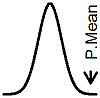P.Mean >> Category >> Poisson regression (created 2007-08-13).

Poisson regression is quite simply a regression model that assumes that the outcome variable follows a Poisson distribution. These regression models are commonly used to predict count or rate variables. These pages describe how Poisson regression works and some of the issues associated with these models. Also see Category: Linear regression, Category: Logistic regression.

2011

11. What is the Poisson distribution? (January/February 2011)

2010

10. P.Mean: Power calculations for comparison of Poisson counts across two groups (created 2010-01-11). Suppose you want to compare Poisson count variables across two groups. How much data would you need to collect? It's a tricky question and there are several approaches that you can consider.

Other resources

Generalized Linear Models. Description: Peter McCullagh and James Nelder wrote the classic reference for the generalized linear model. The generalized linear model is indeed very general, as it includes linear regression, logistic regression, and Poisson regression models as special cases. This book is for students who want more mathematical details.

Modeling Frequency and Count Data. Description: This books presumes that you are already familar with the Generalized Linear Model, and proceeds to show you how to apply these models for a fascinating range of data sets. This book is for students who want more mathematical details.

Elhai JD, Calhoun PS, Ford JD. Statistical procedures for analyzing mental health services data. Psychiatry Research. 2008;160(2):129-136. Available at: http://www.ncbi.nlm.nih.gov/pubmed/18585790 [Accessed May 19, 2009].All of the material above this paragraph is licensed under a Creative Commons Attribution 3.0 United States License. This page was written by Steve Simon and was last modified on 2017-06-15. The material below this paragraph links to my old website, StATS. Although I wrote all of the material listed below, my ex-employer, Children's Mercy Hospital, has claimed copyright ownership of this material. The brief excerpts shown here are included under the fair use provisions of U.S. Copyright laws.

2008

9. Stats: Modeling a declining count variable (June 14, 2008). I've been working on an interesting project that requires Poisson regression. A company sends out a mailing and gets a certain number of telephone calls back on each of the days following. The number of phone calls is typically (but not always) highest on the first day afterwards and declines rapidly on successive days. I wanted to develop a simple Poisson regression model for this data.

8. Stats: Upcoming topics in Poisson regression (April 24, 2008). I get a lot of questions about Poisson regression. I feel embarrassed when this happens because my pages on this topic are woefully incomplete. Everything on my web pages is incomplete to some extent, of course, but this is an area with the biggest gaps. I have been planning for quite a while to write more about this topic, and here are some of the areas I want to discuss.

2007

7. Stats: Confidence interval for a rate (October 10, 2007). Dear Professor Mean, How do you calculate a confidence interval for a rate?

6. Stats: Calculating rates (April 6, 2007). Someone on the MedStats discussion group asked how to calculate a rate of needlestick incidents. The answer is quite simple, but there are a variety of possible responses.

5. Stats: Confidence intervals for count data (March 22, 2007). If you have data involving counts, you have several options for computing confidence intervals. All of these approaches rely on approximations to the Poisson distribution or to relationships involving the Poisson distribution and other important distributions. I want to summarize some of these approaches.

4. Stats: Formulas for cumulative Poisson and binomial probabilities (February 19, 2007). I am updating some material about Poisson regression and noticed that some of the tests and confidence intervals rely on a percentile from a Chi-squared distribution or a gamma distribution. In previous work on binomial confidence intervals, I had noticed the use of a beta distribution and an F distribution. It seems odd to apply percentiles from continuous distributions for confidence intervals involving counting, but the formulas do indeed work. There are well known relationships for the cumulative distributions of the Poisson and binomial distributions that lead to these formulas.

3. Stats: Books that discuss Poisson regression (January 19, 2007). Someone on the MedStats discussion group asked about books discussing Poisson regression. If you want to use Poisson regression, you need an overview of the generalized linear model. The classic reference: Generalized Linear Models. P. McCullagh, J.A. Nelder (1983) London: Chapman and Hall. is quite old, but the book is still worth reading.

2006

2. Stats: Poisson regression? Maybe not! (March 10, 2006). I get a lot of questions about Poisson regression, even though I have very little about it on my web pages. My guess is that there is even less information out there on the rest of the web, so even my meager offerings still place me at the top of the Google search list. I have been wanting to expand my material in this area for quite some time, but just have not had the time. Anyway, someone asked me today if they could use Poisson regression when their outcome variables was the answer to the question "How many children would you like to have?"

1999

1. Stats: Guidelines for poisson regression models (September 21, 1999). Dear Professor Mean, I have just received feedback on a manuscript under review in which one reviewer recommended use of Poisson regression. I am not familiar with this technique--when it is appropriate and/or recommended, what assumptions the data must meet, whether the procedure in SAS? SPSS? I would appreciate a reference and/or citation to article(s) in which it has been used. Thanks! -- Denied Denise

Definitions:

Definition: Poisson distribution

What now?

Browse other categories at this site